# Line and Scatter Plots#

This lesson discusses creating plots using matplotlib’s object oriented interface. “Object oriented” here refers to the fact that when we use this interface, the figure, figure axes and other plot components will be available to use as variables or objects. Using matplotlib this way will allow us to have greater control over our plots.

Let’s see how we can make the same plots we made with the procedural interface using an object oriented approach.

import pandas as pd

import matplotlib.pyplot as plt


s_orbitals.head()

r 1s 2s 3s
0 0.000000 0.564190 0.199471 0.108578
1 0.517241 0.336349 0.114183 0.061683
2 1.034483 0.200519 0.057408 0.029966
3 1.551724 0.119542 0.020580 0.009313
4 2.068966 0.071266 -0.002445 -0.003390
%matplotlib notebook


## Creating Figures and Axes#

Next, we use matplotlib to create an empty figure using the function plt.subplots. This function returns two values - the figure object, and the axis.

fig, ax = plt.subplots()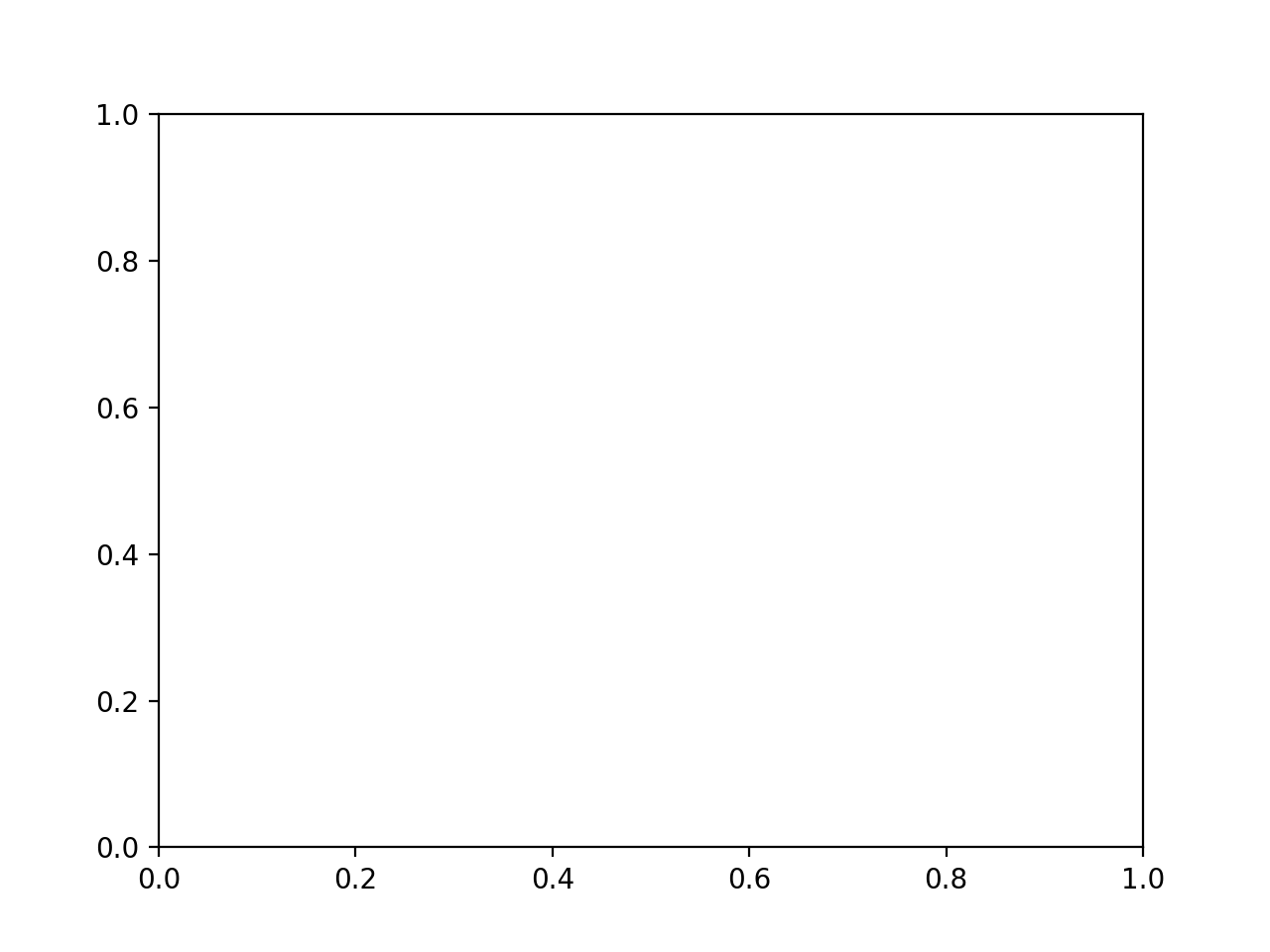You can see a blank figure which matplotlib has created, above. This figure doesn’t have any data yet because we haven’t added it. We will add a line plot data to our figure axis using the command ax.plot. This differs from the procedural interface discussed previously in that it uses the variable ax which was returned from plt.subplots instead of using plt.plot, which is the matplotlib function. In the plot command, you first list the x values to plot, followed by the y values.

fig, ax = plt.subplots()
ax.plot(s_orbitals["r"], s_orbitals["1s"])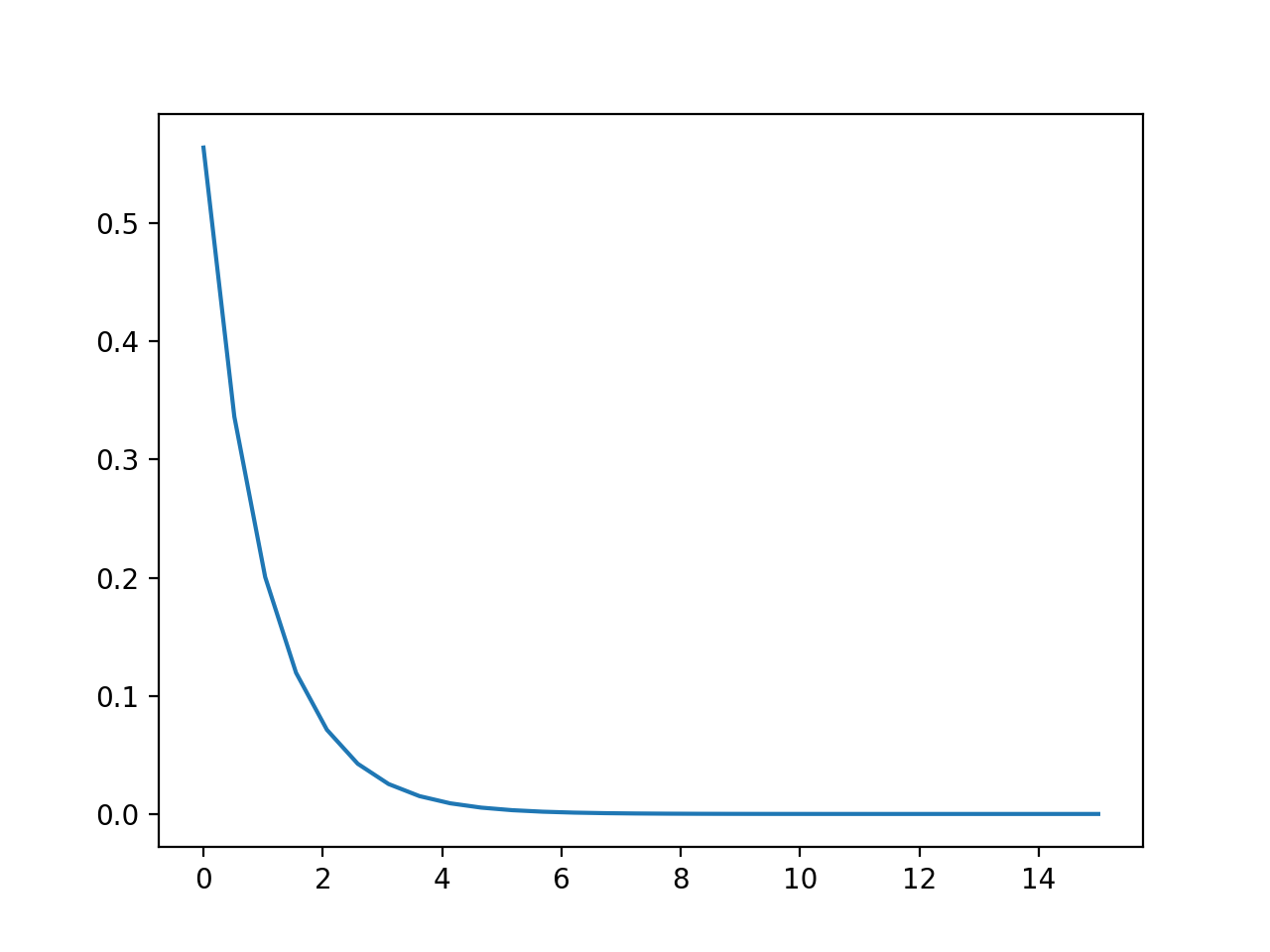[<matplotlib.lines.Line2D at 0x7fb01c041e50>]


When we are using labled data like a pandas dataframe, we can shorten having to type the dataframe variable multiple times by using a different plotting syntax. We will first give the column name which represents x, then the column name which represents y, then we give the dataframe name to the data argument.

fig, ax = plt.subplots()
ax.plot("r", "1s", data=s_orbitals)[<matplotlib.lines.Line2D at 0x7fb01c0aed90>]


You can add additional lines to the figure by adding more plot commands with different data.

fig2, ax2 = plt.subplots()
ax2.plot("r", "1s", data=s_orbitals)
ax2.plot("r", "2s", data=s_orbitals)
ax2.plot("r", "3s", data=s_orbitals)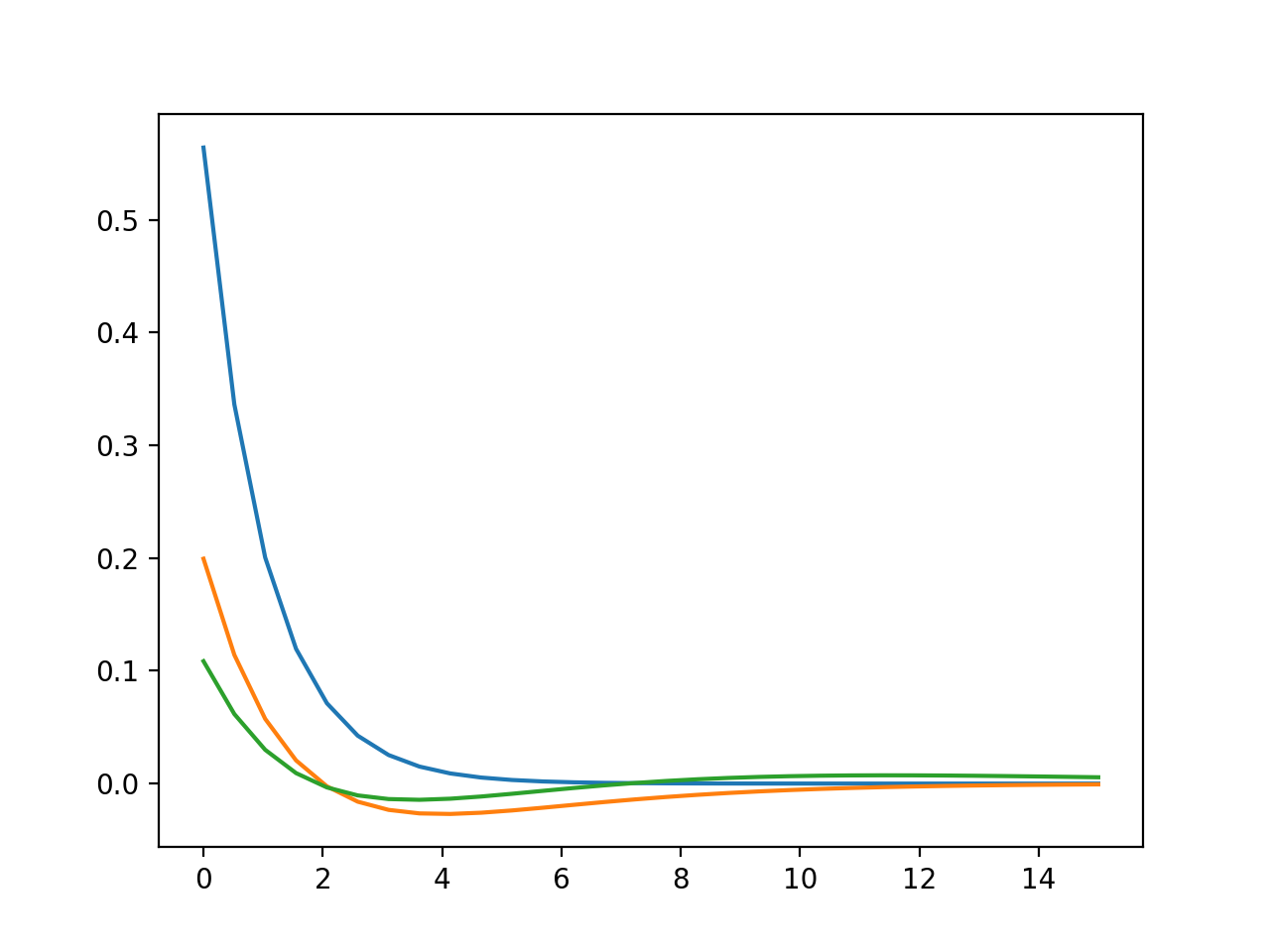[<matplotlib.lines.Line2D at 0x7fb017985d30>]


Note

If you wanted to make a scatter plot with the data instead, you would switch your plot command from ax.plot to ax.scatter. You can even combine ax.scatter with ax.plot in order to get the same data plotted with both. You can try out this example

fig, ax = plt.subplots()
ax.scatter("r", "1s", data=s_orbitals)


The difference between this object oriented and procedural interface is that now we can add or manipulate figures we have already created, as long as they are stored as variables. Try this code to see what happends to Figure 1.


ax.scatter("r", "1s", data=s_orbitals)


## Customizing Plot Appearance#

Matplotlib offers a lot of control over the way your plot appears. We will discuss some topics here including:

1. Setting plot colors

2. Setting labels and legends

3. Setting x and y axis ranges

### Setting Plot Colors#

You can change the color of an individual line by setting the line color property. You can use either a built-in name for the color, or you can specify the hex value. You can find a list of named colors in the matplotlib documentation.

You can use hex values for complete control over colors. For example, we could choose to use MolSSI colors on our plot:

fig, ax = plt.subplots()

ax.plot("r", "1s", data=s_orbitals, color="#2565E8")
ax.plot("r", "2s", data=s_orbitals, color="#ed1c24")
ax.plot("r", "3s", data=s_orbitals, color="#393536")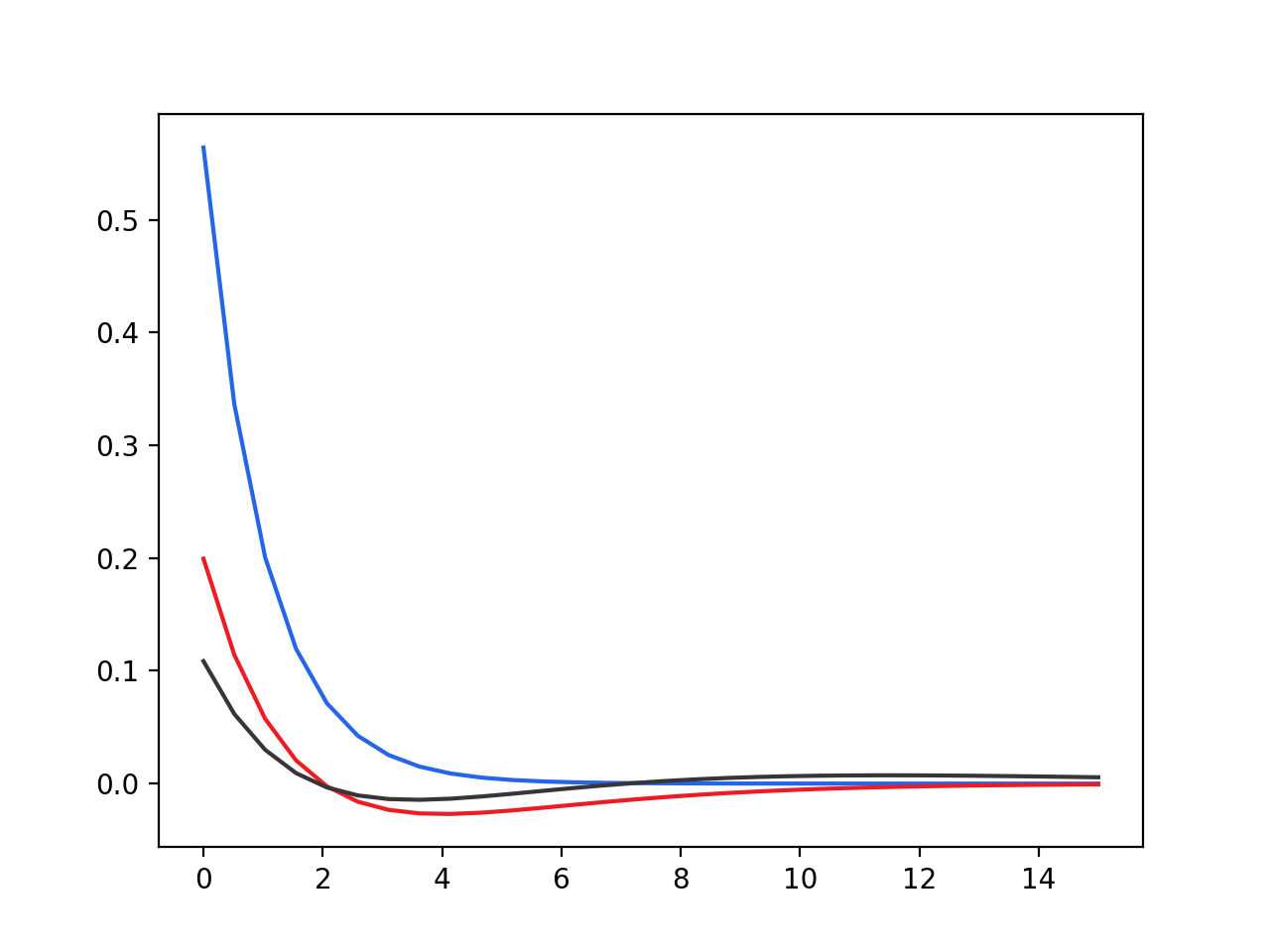[<matplotlib.lines.Line2D at 0x7fb018e08d90>]


### Data Labels and Legends#

We might also want to label our data and have that show up as a legend in the plot. We add another argument called label to the plot command to achieve this. In order for our label to show up, we have to also remember the ax.legend() command.

We might also wish to set a label for the axes. We can use ax.xlabel or ax.ylabel for to display an axis label.

fig, ax = plt.subplots()
ax.plot("r", "1s", data=s_orbitals, color="#2565E8")
ax.plot("r", "2s", data=s_orbitals, color="#ed1c24")
ax.plot("r", "3s", data=s_orbitals, color="#393536")
ax.legend()

ax.set_ylabel("Psi")
ax.set_xlabel("r")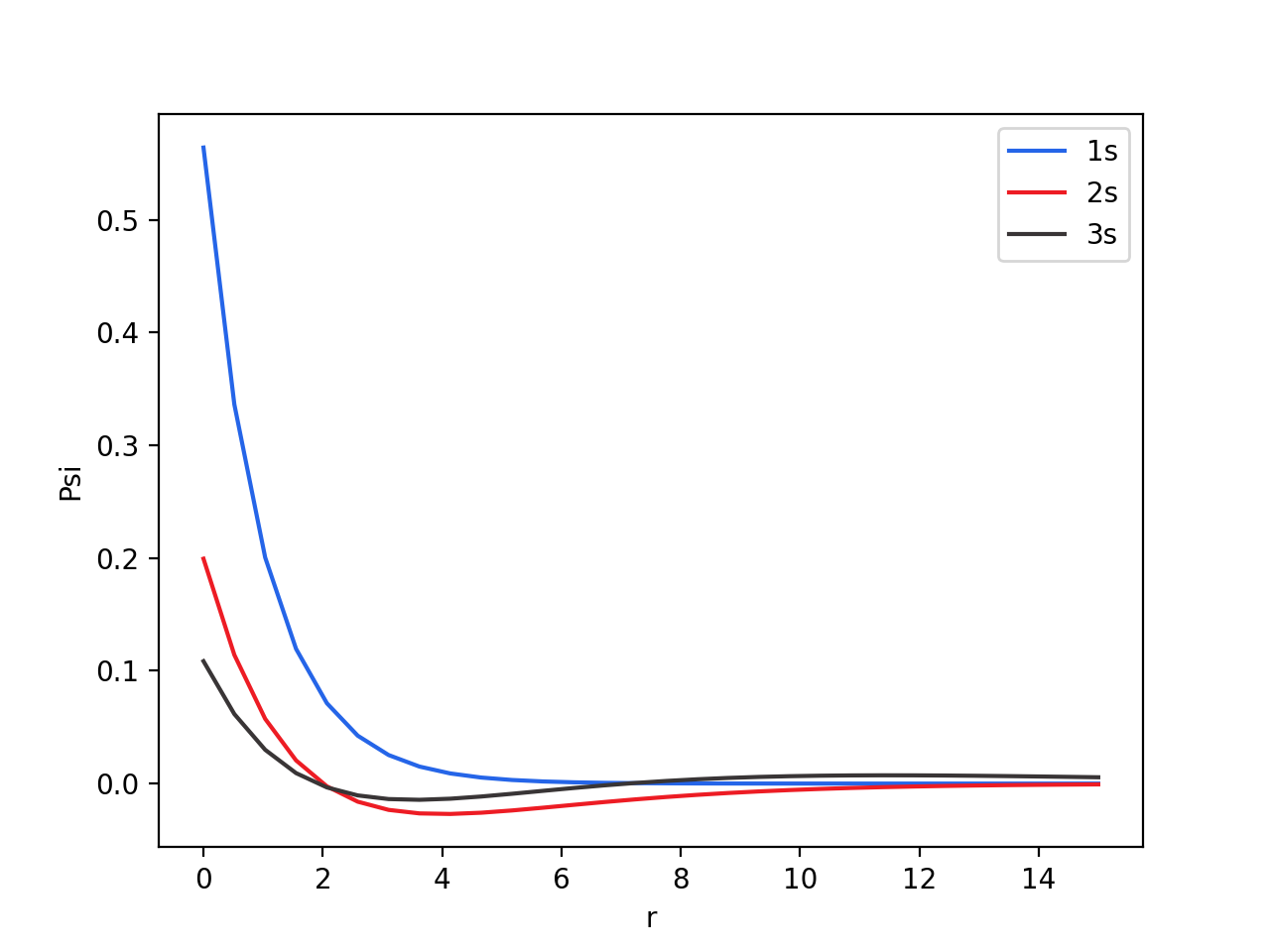Text(0.5, 0, 'r')


Note

When plotting with a pandas dataframe, the column name will automatically be used as the label. If you want to override this, or if you are plotting with something like a numpy array instead, you can add a label to the data when you plot it.

fig, ax = plt.subplots()
ax.plot("r", "1s", data=s_orbitals, color="#2565E8", label="1s orbital")
ax.legend()


Matplotlib supports mathematical expressions in labels as well. We might want to print $$\psi$$ instead of Psi on our y label. To use math text, put an r before your label string, and type your mathematical symbols between dollar signs ($). To use a greek symbol, you do a backward slash followed by the word for the symbol fig, ax = plt.subplots() ax.plot("r", "1s", data=s_orbitals, color="#2565E8") ax.plot("r", "2s", data=s_orbitals, color="#ed1c24") ax.plot("r", "3s", data=s_orbitals, color="#393536") ax.legend() ax.set_ylabel(r"$\psi$") ax.set_xlabel("r")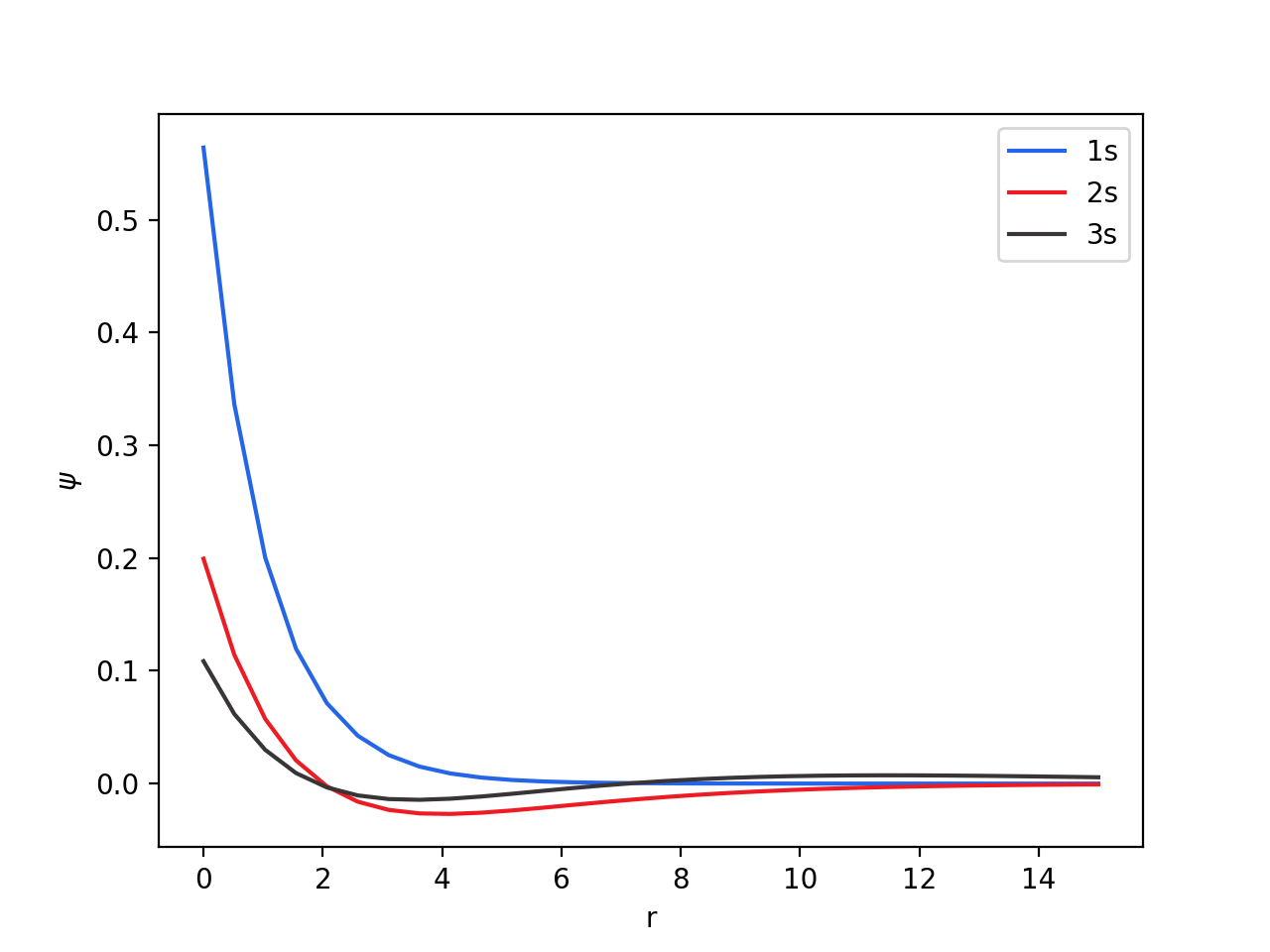Text(0.5, 0, 'r')  Note By default, matplotlib will place the legend where it ‘best’ fits on the plot. You can specify the location, however by adding loc to the legend function. For example, to place the legend in the upper left corner, you would use ax.legend(loc="upper left")  Other locations you can use are ‘upper right’, ‘lower left’, ‘lower right’, ‘right’, ‘center left’, ‘center right’, ‘lower center’, ‘upper center’, ‘center’. ### Setting Axis Ranges# You may have noticed that our plot area shows before and after our data starts. We might wish to set a custom range for axis in order to better see our data. This is accomplished with ax.set_xlim and ax.set_ylim. In the plot below, we set the limits for the x axis, so that it only shows us the plot when x is between 0 and 14. fig, ax = plt.subplots() ax.plot("r", "1s", data=s_orbitals, color="#2565E8") ax.plot("r", "2s", data=s_orbitals, color="#ed1c24") ax.plot("r", "3s", data=s_orbitals, color="#393536") ax.legend() ax.set_ylabel(r"$\psi\$")
ax.set_xlabel("r")
ax.set_xlim(0, 14)